Question

# Review: Square Coil, Round Hole Due this Friday, Mar 29 at 11:59 pm (EDT) A square,...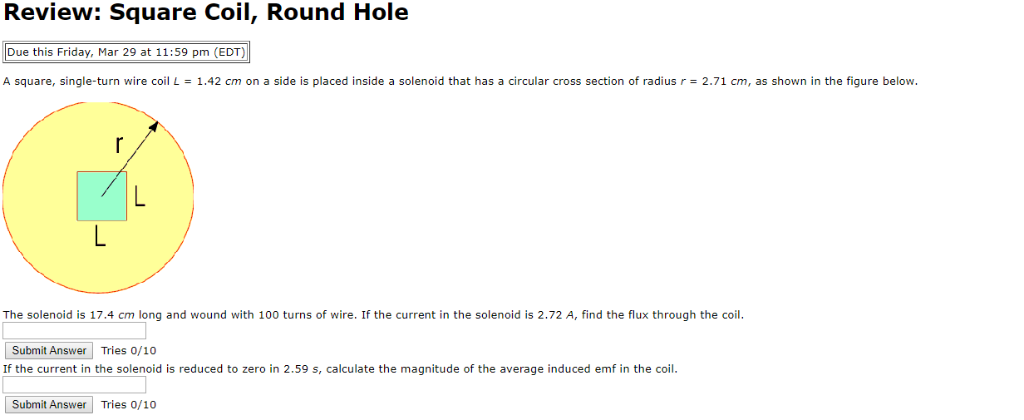Review: Square Coil, Round Hole Due this Friday, Mar 29 at 11:59 pm (EDT) A square, single-turn wire coil L-1.42 cm on a side is placed inside a solenoid that has a circular cross section of radius r 2.71 cm, as shown in the figure below. The solenoid is 17.4 cm long and wound with 100 turns of wire. If the current in the solenoid is 2.72 A, find the flux through the coil Submit Answer Tries 0/10 If the current in the solenoid is reduced to zero in 2.59 s, calculate the magnitude of the average induced emf in the coil. Submit Answer Tries 0/10

a)

flux through the loop is given by

phi = BA = ( uo N i/L) A

phi = 4* 3.14* 10^-7* 100* 2.72 * (1.42*10^-2)^2 / (0.174)

phi = 3.96*10^-7 Tm^2

======

b)

emf induced

e = change in flux / time

e = (phi - 0) / t

e = 3.96*10^-7 / 2.59

e = 1.529*10^-7 V

=======

#### Earn Coins

Coins can be redeemed for fabulous gifts.

Similar Homework Help Questions
• ### Square Coil, Round Hole Due this Friday, Feb 23 at 11:59 pm (EST) A square, single-turn...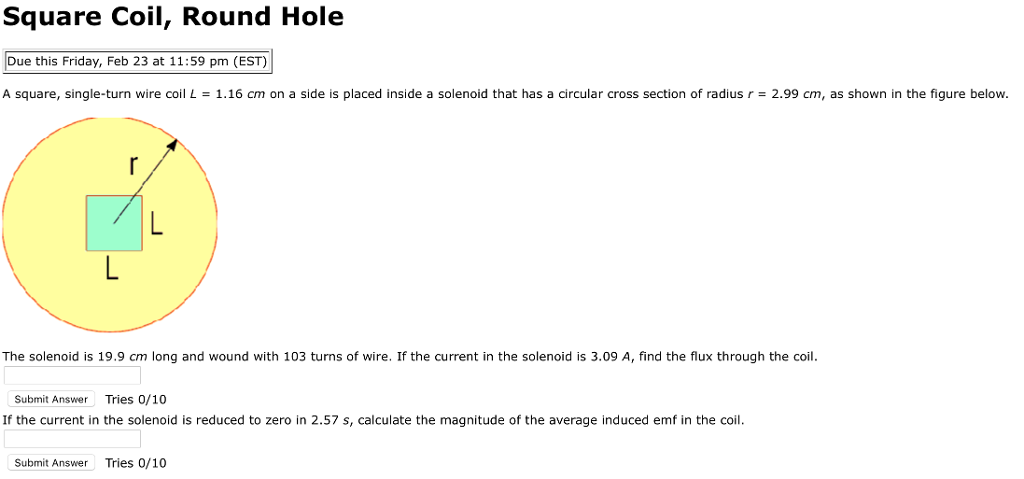Square Coil, Round Hole Due this Friday, Feb 23 at 11:59 pm (EST) A square, single-turn wire coil L = 1.16 cm on a side is placed inside a solenoid that has a circular cross section of radius r = 2.99 cm, as shown in the figure below The solenoid is 19.9 cm long and wound with 103 turns of wire. If the current in the solenoid is 3.09 A, find the flux through the coil Submit Answer Tries 0/10...

• ### A square, single-turn wire coil L = 1.52 cm on a side is placedinside a...

A square, single-turn wire coil L = 1.52 cm on a side is placed inside a solenoid that has a circular cross-section of radius r = 2.79 cm, as shown in the figure below.The solenoid is 18.0 cm long and wound with 107 turns of wire. If the current in the solenoid is 3.38 A, find the flux through the coil.If the current in the solenoid is reduced to zero in 3.37 s, calculate the magnitude of the average induced...

• ### Semicircles of current Due this Wednesday, Mar 25 at 11:59 pm (EDT) A current is set...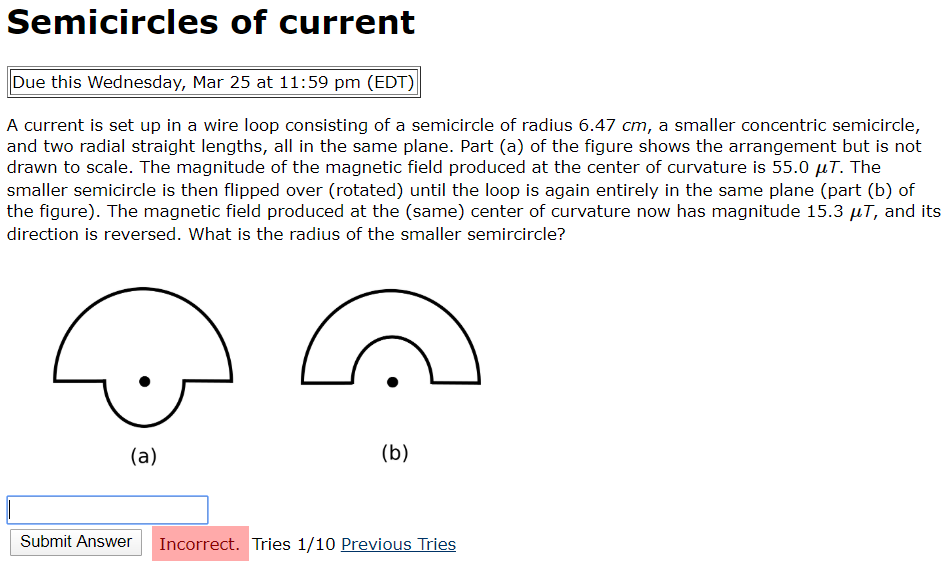Semicircles of current Due this Wednesday, Mar 25 at 11:59 pm (EDT) A current is set up in a wire loop consisting of a semicircle of radius 6.47 cm, a smaller concentric semicircle, and two radial straight lengths, all in the same plane. Part (a) of the figure shows the arrangement but is not drawn to scale. The magnitude of the magnetic field produced at the center of curvature is 55.0 UT. The smaller semicircle is then flipped over (rotated)...

• ### Prescribing Eyeglasses - Hyperopia Due this Friday, Mar 30 at 11:59 pm (EDT) Suppose you have...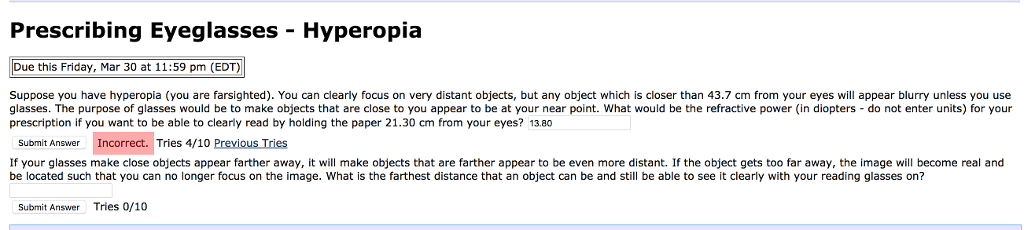Prescribing Eyeglasses - Hyperopia Due this Friday, Mar 30 at 11:59 pm (EDT) Suppose you have hyperopia (you are farsighted). You can clearly focus on very distant objects, but any object which is closer than 43.7 cm from your eyes will appear blurry unless you use glasses. The purpose of glasses would be to make objects that are close to you appear to be at your near point. What would be the refractive power (in diopters - do not enter...

• ### A square, single-turn wire loop ℓ = 1.00 cm on a side is placed inside a...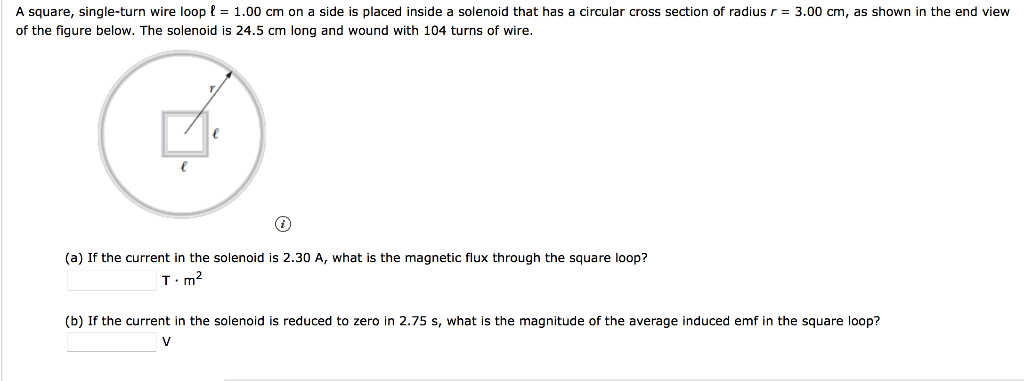A square, single-turn wire loop ℓ = 1.00 cm on a side is placed inside a solenoid that has a circular cross section of radius r = 3.00 cm, as shown in the end view of the figure below. The solenoid is 24.5 cm long and wound with 104 turns of wire. (a) If the current in the solenoid is 2.30 A, what is the magnetic flux through the square loop? T · m2 (b) If the current in the...

• ### Block and Spring irn SHM with damping Due this Friday, Apr 20 at 11:59 pm (EDT)...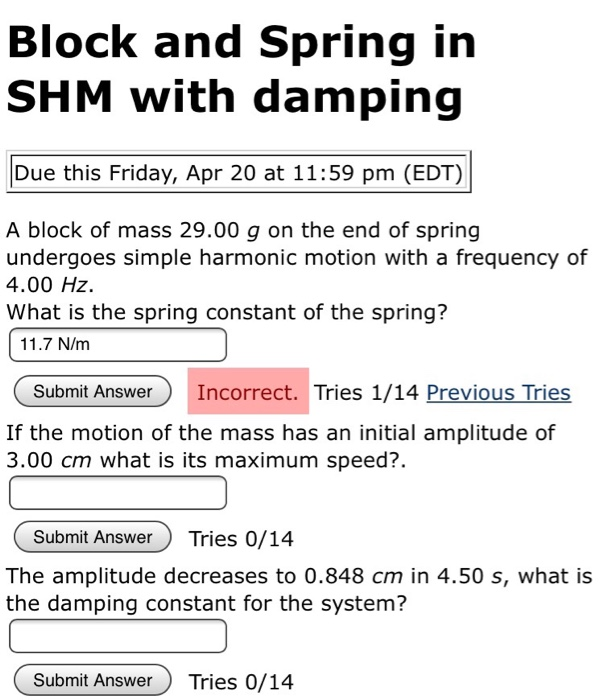Block and Spring irn SHM with damping Due this Friday, Apr 20 at 11:59 pm (EDT) A block of mass 29.00 g on the end of spring undergoes simple harmonic motion with a frequency of 4.00 HZ What is the spring constant of the spring? 11.7 N/m Submit AnswerIncorrect. Tries 1/14 Previous Tries If the motion of the mass has an initial amplitude of 3.00 cm what is its maximum speed?. Submit Answer Tries 0/14 The amplitude decreases to 0.848...

• ### Block and Spring in SHM with damping Due this Friday, Apr 19 at 11:59 pm (EDT)...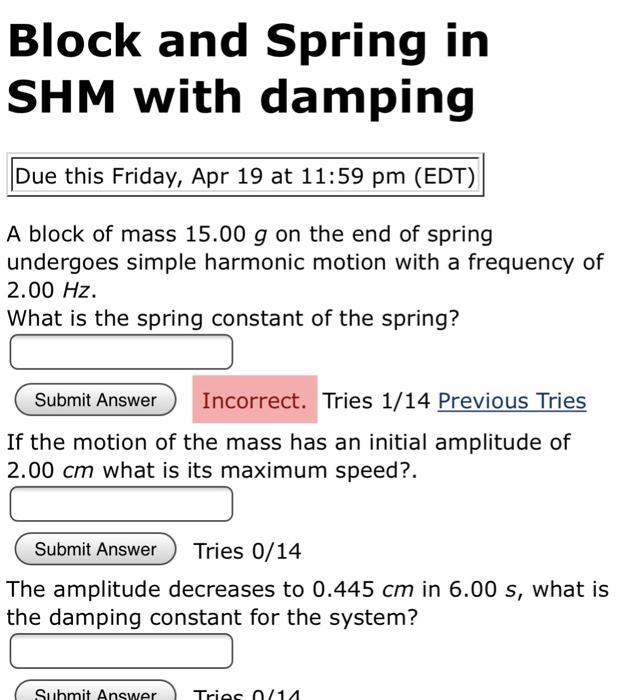Block and Spring in SHM with damping Due this Friday, Apr 19 at 11:59 pm (EDT) A block of mass 15.00 g on the end of spring undergoes simple harmonic motion with a frequency of 2.00 Hz. What is the spring constant of the spring? Submit Answer Incorrect. Tries 1/14 Previous Tries If the motion of the mass has an initial amplitude of 2.00 cm what is its maximum speed?. Submit AnswerTries 0/14 The amplitude decreases to 0.445 cm in...

• ### Please solve for part A,B and C Review I Constants A solenoidal coil with 30 turns...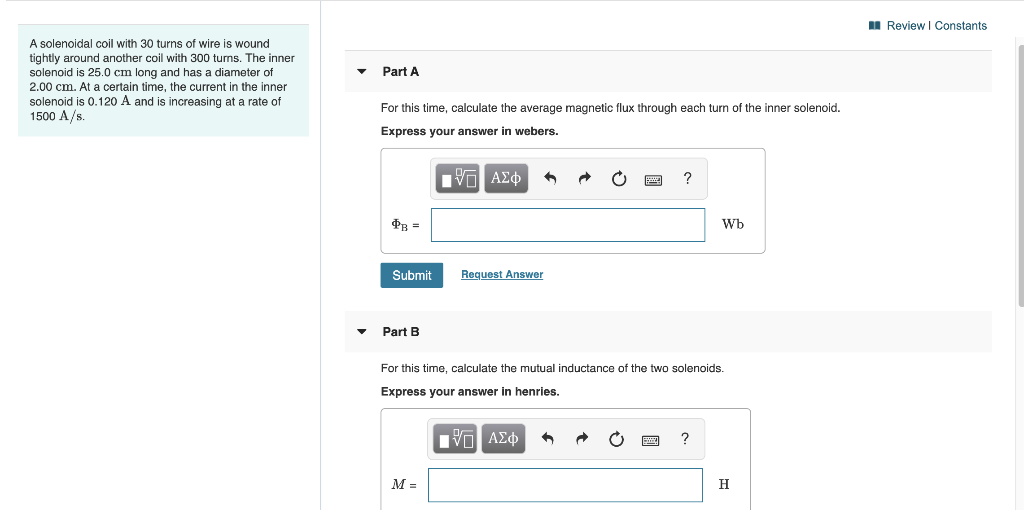Please solve for part A,B and C Review I Constants A solenoidal coil with 30 turns of wire is wound tightly around another coil with 300 turns. The inner solenoid is 25.0 cm long and has a diameter of 2.00 cm. At a certain time, the current in the inner solenoid is 0.120 A and is increasing at a rate of 1500 A/s Part A For this time, calculate the average magnetic flux through each turn of the inner solenoid....

• ### A solenoidal coil with 30 turns of wire is wound tightly around another coil with 350...A solenoidal coil with 30 turns of wire is wound tightly around another coil with 350 turns. The inner solenoid is 23.0 cm long and has a Part A diameter of 2.10 cm. At a certain time, the current in the inner solenoid is 0.120 A and is increasing at a rate of 1800 A/s. For this time, calculate the average magnetic flux through each turn of the inner solenoid. Express your answer in webers. Vη ΑΣφ' Фв - Wb...

• ### Part A A solenoidal coil with 25 turns of wire is wound tightly around another coil...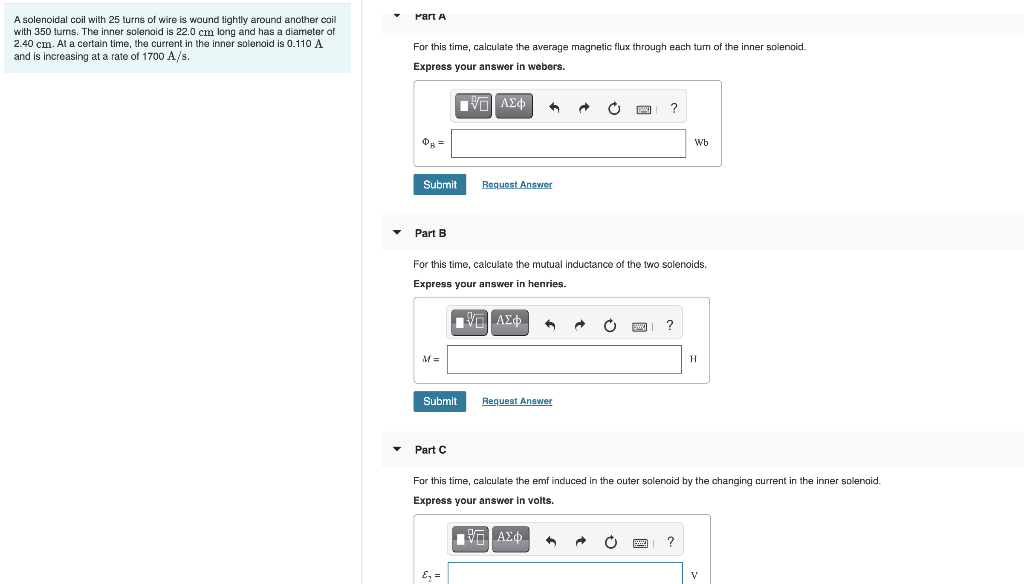Part A A solenoidal coil with 25 turns of wire is wound tightly around another coil with 350 turns. The inner solenoid is 22.0 cm long and has a diameter of 2.40 cm. At a certain time, the current in the inner solenoid is 0.110 A and is increasing at a rate of 1700 A/s. For this time, calculate the average magnetic flux through each turn of the inner solenoid. Express your answer in webers. Ivo A20 ? Wb Submit...